International
Tables for
Crystallography
Volume A
Space-group symmetry
Edited by M. I. Aroyo

International Tables for Crystallography (2016). Vol. A, ch. 1.2, pp. 19-21

Section 1.2.3. Symmetry elements

H. Wondratscheka and M. I. Aroyob

aLaboratorium für Applikationen der Synchrotronstrahlung (LAS), Universität Karlsruhe, Germany, and bDepartamento de Física de la Materia Condensada, Universidad del País Vasco (UPV/EHU), Bilbao, Spain

1.2.3. Symmetry elements

| top | pdf |

In the 1970s, when the International Union of Crystallography (IUCr) planned a new series of International Tables for Crystallography to replace the series International Tables for X-ray Crystallography (1952), there was some confusion about the use of the term symmetry element. Crystallographers and mineralogists had used this term for rotation and rotoinversion axes and reflection planes, in particular for the description of the morphology of crystals, for a long time, although there had been no strict definition of symmetry element'. With the impact of mathematical group theory in crystallography the term element was introduced with another meaning, in which an element is a member of a set, in particular as a group element of a group. In crystallography these group elements, however, were the symmetry operations of the symmetry groups, not the crystallographic symmetry elements. Therefore, the IUCr Commission on Crystallographic Nomenclature appointed an Ad-hoc Committee on the Nomenclature of Symmetry with P. M. de Wolff as Chairman to propose definitions for terms of crystallographic symmetry and for several classifications of crystallographic space groups and point groups.

In the reports of the Ad-hoc Committee, de Wolff et al. (1989) and (1992) with Addenda, Flack et al. (2000), the results were published. To define the term symmetry element for any symmetry operation was more complicated than had been envisaged previously, in particular for unusual screw and glide components.

According to the proposals of the Committee the following procedure has been adopted (cf. also Table 1.2.3.1):

• (1) No symmetry element is defined for the identity and the (lattice) translations.

 Table 1.2.3.1| top | pdf | Symmetry elements in point and space groups
Name of symmetry elementGeometric elementDefining operation (d.o.)Operations in element set
Mirror plane Plane p Reflection through p D.o. and its coplanar equivalents
Glide plane Plane p Glide reflection through p; 2v (not v) a lattice-translation vector D.o. and its coplanar equivalents
Rotation axis Line l Rotation around l, angle 2π/N, N = 2, 3, 4 or 6 1st … (N − 1)th powers of d.o. and their coaxial equivalents
Screw axis Line l Screw rotation around l, angle 2π/N, u = j/N times shortest lattice translation along l, right-hand screw, N = 2, 3, 4 or 6, j = 1, …, (N − 1) 1st … (N − 1)th powers of d.o. and their coaxial equivalents
Rotoinversion axis Line l and point P on l Rotoinversion: rotation around l, angle 2π/N, followed by inversion through P, N = 3, 4 or 6 D.o. and its inverse
Centre Point P Inversion through P D.o. only
That is, all glide reflections through the same reflection plane, with glide vectors v differing from that of the d.o. (taken to be zero for reflections) by a lattice-translation vector. The glide planes a, b, c, n, d and e are distinguished (cf. Table 2.1.2.1).
That is, all rotations and screw rotations around the same axis l, with the same angle and sense of rotation and the same screw vector u (zero for rotation) up to a lattice-translation vector.
• (2) For any symmetry operation of point groups and space groups with the exception of the rotoinversions,and, the geometric element is defined as the set of fixed points (the second column of Table 1.2.3.1) of the reduced operation, cf. equation (1.2.2.17). For reflections and glide reflections this is a plane; for rotations and screw rotations it is a line, for the inversion it is a point. For the rotoinversions,andthe geometric element is a line with a point (the inversion centre) on this line.

• (3) The element set (cf. the last column of Table 1.2.3.1) is defined as a set of operations that share the same geometric element. The element set can consist of symmetry operations of the same type (such as the powers of a rotation) or of different types, e.g. by a reflection and a glide reflection through the same plane. The defining operation (d.o.) may be any symmetry operation from the element set that suffices to identify the symmetry element. In most cases, the simplest' symmetry operation from the element set is chosen as the d.o. (cf. the third column of Table 1.2.3.1). For reflections and glide reflections the element set includes the defining operation and all glide reflections through the same reflection plane but with glide vectors differing by a lattice-translation vector, i.e. the so-called coplanar equivalents. For rotations and screw rotations of angle 2π/k the element set is the defining operation, its 1st … (k − 1)th powers and all rotations and screw rotations with screw vectors differing from that of the defining operation by a lattice-translation vector, known as coaxial equivalents. For a rotoinversion the element set includes the defining operation and its inverse.

• (4) The combination of the geometric element and its element set is indicated by the name symmetry element. The names of the symmetry elements (first column of Table 1.2.3.1) are combinations of the name of the defining operation attached to the name of the corresponding geometric element. Names of symmetry elements are mirror plane, glide plane, rotation axis, screw axis, rotoinversion axis and centre.2 This allows such statements as this point lies on a rotation axis or these operations belong to a glide plane.

Examples

• (1) Glide and mirror planes. The element set of a glide plane with a glide vector v consists of infinitely many different glide reflections with glide vectors that are obtained from v by adding any lattice-translation vector parallel to the glide plane, including centring translations of centred cells.

 (a) It is important to note that if among the infinitely many glide reflections of the element set of the same plane there exists one operation with zero glide vector, then this operation is taken as the defining operation (d.o). Consider, for example, the symmetry operation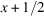,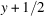,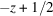of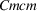(63) [General position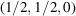block]. This is an n-glide reflection through the plane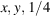. However, the corresponding symmetry element is a mirror plane, as among the glide reflections of the element set of the planeone finds the reflection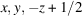[symmetry operation (6) of the General positionblock]. (b) The symmetry operation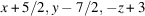is a glide reflection. Its geometric element is the plane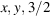. Its symmetry element is a glide plane in space group Pmmn (59) because there is no lattice translation by which the glide vector can be changed to o. If, however, the same mapping is a symmetry operation of space group Cmmm (65), then its symmetry element is a reflection plane because the glide vector with components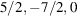can be cancelled through a translation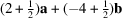, which is a lattice translation in a C lattice. Evidently, the correct specification of the symmetry element is possible only with respect to a specific translation lattice. (c) Similarly, in Cmme (67) with an a-glide reflection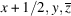, the b-glide reflection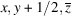also occurs. The geometric element is the planeand the symmetry element is an e-glide plane. In fact, all vectors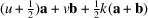,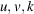integers, are glide vectors of glide reflections through the (001) plane of a space group with a C-centred lattice. Among them one finds a glide reflection b with a glide vector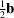related to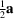by the centring translation; an a-glide reflection and a b-glide reflection share the same plane as a geometric element. Their symmetry element is thus an e-glide plane. (d) In general, the e-glide planes are symmetry elements characterized by the existence of two glide reflections through the same plane with perpendicular glide vectors and with the additional requirement that at least one glide vector is along a crystal axis (de Wolff et al., 1992). The e-glide designation of glide planes occurs only when a centred cell represents the choice of basis (cf. Table 2.1.2.2). The `double' e-glide planes are indicated by special graphical symbols on the symmetry-element diagrams of the space groups (cf. Tables 2.1.2.3and 2.1.2.4). For example, consider the space group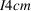(108). The symmetry operations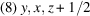[General position (0, 0, 0) block] and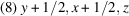[General position (1/2, 1/2, 1/2) block] are glide reflections through the same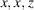plane, and their glide vectors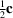and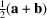are related by the centring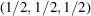translation. The corresponding symmetry element is an e-glide plane and it is easily recognized on the symmetry-element diagram ofshown in Chapter 2.3.

• (2) Screw and rotation axes. The element set of a screw axis is formed by a screw rotation of angle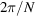with a screw vector u, its (N − 1) powers and all its co-axial equivalents, i.e. screw rotations around the same axis, with the same angle and sense of rotation, with screw vectors obtained by adding a lattice-translation vector parallel to u.

 (a) Twofold screw axis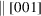in a primitive cell: the element set is formed by all twofold screw rotations around the same axis with screw vectors of the type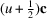, i.e. screw components as,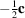,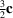etc. (b) The symmetry operation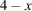,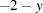,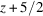is a screw rotation of space group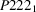(17). Its geometric element is the line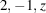and its symmetry element is a screw axis. (c) The determination of the complete element set of a geometric element is important for the correct designation of the corresponding symmetry element. For example, the symmetry element of a twofold screw rotation with an axis through the origin is a twofold screw axis in the space groupbut a fourfold screw axis in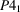(76).

• (3) Special case. In point groups,and space groups(175),(191) and(192) the geometric elements of the defining operationsandare the same. To make the element sets unique, the geometric elements should not be given just by a line and a point on it, but should be labelled by these operations. Then the element sets and thus the symmetry element are unique (Flack et al., 2000).

References

International Tables for X-ray Crystallography (1952). Vol. I, edited by N. F. M. Henry & K. Lonsdale. Birmingham: Kynoch Press.
Flack, H. D., Wondratschek, H., Hahn, Th. & Abrahams, S. C. (2000). Symmetry elements in space groups and point groups. Addenda to two IUCr Reports on the Nomenclature of Symmetry. Acta Cryst. A56, 96–98.
Wolff, P. M. de, Billiet, Y., Donnay, J. D. H., Fischer, W., Galiulin, R. B., Glazer, A. M., Senechal, M., Shoemaker, D. P., Wondratschek, H., Hahn, Th., Wilson, A. J. C. & Abrahams, S. C. (1989). Definition of symmetry elements in space groups and point groups. Report of the International Union of Crystallography Ad-Hoc Committee on the Nomenclature of Symmetry. Acta Cryst. A45, 494–499.
Wolff, P. M. de, Billiet, Y., Donnay, J. D. H., Fischer, W., Galiulin, R. B., Glazer, A. M., Hahn, Th., Senechal, M., Shoemaker, D. P., Wondratschek, H., Wilson, A. J. C. & Abrahams, S. C. (1992). Symbols for symmetry elements and symmetry operations. Final Report of the International Union of Crystallography Ad-Hoc Committee on the Nomenclature of Symmetry. Acta Cryst. A48, 727–732.